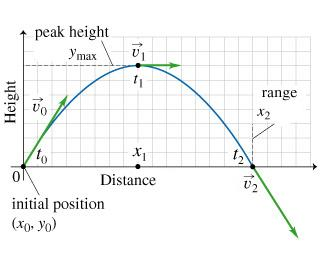# Problem: The figure shows the trajectory of a golf ball in projectile motion over level ground. The time t0 = 0 s corresponds to the moment just after the ball is launched from position x0 = 0 m, y0 = 0 m. Its initial velocity (launch velocity) is v0. Two other points along the trajectory are indicated in the figure. At time t1 (with velocity v1), the ball reaches the peak of its trajectory. Its position at this moment is denoted by (x1,y1) or (x1,ymax), since it is at its maximum height. At time t2 (with velocity v2), just before the ball touches the ground again, its position is (x2,y2) or (xmax,y2), since it is at its maximum horizontal range.How do the speeds v0, v1, and v2 (at times t0, t1, and t2) compare?

###### FREE Expert Solution

The magnitudes of v0 and v2 are the same. Speed does not include the direction.

85% (335 ratings)###### Problem Details

The figure shows the trajectory of a golf ball in projectile motion over level ground. The time t0 = 0 s corresponds to the moment just after the ball is launched from position x0 = 0 m, y0 = 0 m. Its initial velocity (launch velocity) is v0. Two other points along the trajectory are indicated in the figure. At time t1 (with velocity v1), the ball reaches the peak of its trajectory. Its position at this moment is denoted by (x1,y1) or (x1,ymax), since it is at its maximum height. At time t2 (with velocity v2), just before the ball touches the ground again, its position is (x2,y2) or (xmax,y2), since it is at its maximum horizontal range.How do the speeds v0, v1, and v2 (at times t0, t1, and t2) compare?